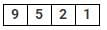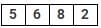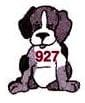Test: Numbers - 1

# Test: Numbers - 1

Test Description

## 10 Questions MCQ Test Mathematics for Class 3: NCERT | Test: Numbers - 1

Test: Numbers - 1 for Class 3 2023 is part of Mathematics for Class 3: NCERT preparation. The Test: Numbers - 1 questions and answers have been prepared according to the Class 3 exam syllabus.The Test: Numbers - 1 MCQs are made for Class 3 2023 Exam. Find important definitions, questions, notes, meanings, examples, exercises, MCQs and online tests for Test: Numbers - 1 below.
Solutions of Test: Numbers - 1 questions in English are available as part of our Mathematics for Class 3: NCERT for Class 3 & Test: Numbers - 1 solutions in Hindi for Mathematics for Class 3: NCERT course. Download more important topics, notes, lectures and mock test series for Class 3 Exam by signing up for free. Attempt Test: Numbers - 1 | 10 questions in 20 minutes | Mock test for Class 3 preparation | Free important questions MCQ to study Mathematics for Class 3: NCERT for Class 3 Exam | Download free PDF with solutions
 1 Crore+ students have signed up on EduRev. Have you?
Test: Numbers - 1 - Question 1

### Sneha had a collection of 948 stickers. Which of he following is the CORRECT number name for 948?

Detailed Solution for Test: Numbers - 1 - Question 1

Number name for 948 is nine hundred and forty eight.

Test: Numbers - 1 - Question 2

### Which of the following shows CORRECT number name of Mr Sharma's house number? Mr Sharma's House NumberDetailed Solution for Test: Numbers - 1 - Question 2

Number name for 9521 is Nine thousand five hundred and twenty one.

Test: Numbers - 1 - Question 3

### Which of the following set of numbers is in the order from the lowest to the highest?

Detailed Solution for Test: Numbers - 1 - Question 3

1920 < 9102 < 9321 < 9468 < 9768
are arranged from the lowest to the highest.

Test: Numbers - 1 - Question 4

The greatest four digit even number that can be formed using 7, 0, 6, 5 without repeating the digits is __.

Detailed Solution for Test: Numbers - 1 - Question 4

The greatest four digit even number formed by using 7, 0, 6, 5 without repetition is 7650.

Test: Numbers - 1 - Question 5

Which place value will determine the larger number among the two? 7295, 7259

Detailed Solution for Test: Numbers - 1 - Question 5

Here, ten's place determines the larger of the two numbers because both the numbers have same digits at hundred's and thousand's place.

Test: Numbers - 1 - Question 6

How many four digit numbers can be formed, sing 7, 5, 0, 2 only once in a number?

Detailed Solution for Test: Numbers - 1 - Question 6

The four digit numbers formed by using 7, 5, 0, 2 are 2057, 2075, 2507, 2570, 2750, 2705, 5027, 5072, 5702, 5720, 5270, 5207, 7205, 7025, 7250, 7520, 7502, 7052 So, total numbers formed = 18.

Test: Numbers - 1 - Question 7

6935 is same as __.

Detailed Solution for Test: Numbers - 1 - Question 7

6935 = 6000 + 900 + 30 + 5

Test: Numbers - 1 - Question 8

Sonia is playing with some number cards. She made a deck of these cards and pulled the cards as shown below.Her mother asked which is the largest possible 4 digit number can be formed using all cards? Help her to find the answer.

Detailed Solution for Test: Numbers - 1 - Question 8

The largest possible four digit number formed using all cards is 8652.

Test: Numbers - 1 - Question 9

Which of the given abacus shows the number less than 1345?

Detailed Solution for Test: Numbers - 1 - Question 9

Abacus in option (a) shows 1345. Abacus in option (b) shows 5231. Abacus in option (c) shows 1353. Abacus in option (d) shows 1215. So, abacus in option (d) represents the number less than 1345.

Test: Numbers - 1 - Question 10

Which of the following number names belongs to the dog's number?Detailed Solution for Test: Numbers - 1 - Question 10

Nine hundred and twenty seven belongs to the dog's number.

## Mathematics for Class 3: NCERT

52 videos|84 docs|44 tests
 Use Code STAYHOME200 and get INR 200 additional OFF Use Coupon Code
Information about Test: Numbers - 1 Page
In this test you can find the Exam questions for Test: Numbers - 1 solved & explained in the simplest way possible. Besides giving Questions and answers for Test: Numbers - 1, EduRev gives you an ample number of Online tests for practice

## Mathematics for Class 3: NCERT

52 videos|84 docs|44 tests